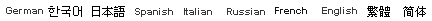﻿ 面积单位换算表翻译-广州翻译公司-深圳翻译公司翻译范围 翻译报价 成功案例 付款方式 人才招聘 翻译须知 翻译流程 联系方式

 您现在的位置：翻译 -翻译公司>> 面积单位换算表
 新译通翻译公司---上海,北京,广州,深圳

 英美制到公制换算 公制到英制换算 Linear Measure 长度单位: 1 inch 英寸=25.4 millimetres 毫米 1 foot 英尺=12 inches 英寸=0.3048 metre 米 1 yard 码=3 feet 英尺=0.9144 metre 米 1 (statute) mile 英里=1760 yards 码 =1.609 kilometres 千米 1 nautical mile 海里=1852 m. 米 Square Measure 面积单位: 1 square inch 平方英寸=6.45 sq.centimetres 平方厘米 1 square foot 平方英尺=144 sq.in.平方英寸 =9.29 sq.decimetres 平方分米 1 square yard 平方码=9 sq.ft. 平方英尺 =0.836 sq.metre 平方米 1 acre 英亩=4840 sq.yd.平方码=0.405 hectare 公顷 1 square mile 平方英里=640 acres 英亩 =259 hectares 公顷 Cubic Measure 体积单位: 1 cubic inch 立方英寸=16.4 cu.centimetres 立方厘米 1 cubic foot 立方英尺=1728 cu.in. 立方英寸 =0.0283 cu.metre 立方米 1 cubic yard 立方码=27 cu.ft. 立方英尺 =0.765 cu.metre 立方米 Capacity Measure 容积单位: Britich 英制 1 pint 品脱=20 fluid oz. 液量盎司 =34.68 cu.in. 立方英寸=0.568 litre 升 1 quart 夸脱=2 pints 品脱=1.136 litres 升 1 gallon 加伦=4 quarts 夸脱=4.546 litres 升 1 peck 配克=2 gallons 加伦=9.092 litres 升 1 bushel 蒲式耳=4 pecks 配克=36.4 litres 升 1 quarter 八蒲式耳=8 bushels 蒲式耳 =2.91 hectolitres 百升 American dry 美制干量 1 pint 品脱=33.60 cu.in. 立方英寸=0.550 litre 升 1 quart 夸脱=2 pints 品脱=1.101 litres 升 1 peck 配克=8 quarts 夸脱=8.81 litres 升 1 bushel 蒲式耳=4 pecks 配克=35.3 litres 升 American liquid 美制液量 1 pint 品脱=16 fluid oz. 液量盎司 =28.88 cu.in. 立方英寸=0.473 litre 升 1 quart 夸脱=2 pints 品脱=0.946 litre 升 1 gallon 加伦=4 quarts 夸脱=3.785 litres 升 Avoirdupois Weight 常衡单位: 1 grain 格令=0.065 gram 克 1 dram 打兰=1.772 grams 克 1 ounce 盎司=16 drams 打兰=28.35 grams 克 1 pound 磅=16 ounces 盎司=7000 grains 谷 =0.4536 kilogram 千克 1 stone 英石=14 pounds 磅=6.35 kilograms 千克 1 quarter 四分之一英担=2 stones 英石 =12.70 kilograms 千克 1 hundredweight 英担=4 quarters 四分之一英担 =50.80 kilograms 千克 1 short ton 短吨(美吨)=2000 pounds 磅 =0.907 tonne 公吨 1 (long) ton 长吨(英吨)=20 hundredweight 英担 =1.016 tonnes 公吨 Linear Measure 长度单位: 1 millimetre 毫米=0.03937 inch 英寸 1 centimetre 厘米=10 mm. 毫米=0.3937 inch 英寸 1 decimetre 分米=10 cm. 厘米=3.937 inches 英寸 1 metre 米=10 dm. 分米=1.0936 yards 码=3.2808 feet 英尺 1 decametre 十米=10 m. 米=10.936 yards 码 1 hectometre 百米=100 m. 米=109.4 yards 码 1 kilometre 千米=1000 m. 米=0.6214 mile 英里 1 mile marin 海里=1852 m. 米=1.1500 mile 英里 Square Measure 面积单位: 1 square centimetre 平方厘米=0.155 sq.inch 平方英寸 1 square metre 平方米=1.196 sq.yards 平方码 1 are 公亩=100 square metres 平方米 =119.6 sq.yards 平方码 1 hectare 公顷=100 ares 公亩=2.471 acres 英亩 1 square kilometre 平方公里=0.386 sq.mile 平方英里 Cubic Measure 体积单位: 1 cubic centimetre 立方厘米=0.061 cu.inch 立方英寸 1 cubic metre 立方米=1.308 cu.yards 立方码 Capacity Measure 容积 1 millilitre 毫升=0.002 pint (British) 英制品脱 1 centilitre 厘升=10 ml. 毫升=0.018 pint 品脱 1 decilitre 分升=10 cl. 厘升=0.176 pint 品脱 1 litre 升=10 dl. 分升=1.76 pints 品脱 1 decalitre 十升=10 l. 升=2.20 gallons 加伦 1 hectolitre 百升=100 l. 升=2.75 bushels 蒲式耳 1 kilolitre 千升=1000 l. 升=3.44 quarters 八蒲式耳 Weight 重量单位: 1 milligram 毫克=0.015 grain 谷 1 centigram 厘克=10 mg. 毫克=0.154 grain 谷 1 decigram 分克=10 cg. 厘克=1.543 grains 谷 1 gram 克=10 dg. 分克=15.43 grains 谷 1 decagram 十克=10 g. 克=5.64 drams 打兰 1 hectogram 百克=100 g. 克=3.527 ounces 盎司 1 kilogram 千克=1000 g. 克=2.205 pounds 磅 1 ton (metric ton) 吨,公吨=1000 kg. 千克 =0.984 (long) ton 长吨,英吨=1.1023 短吨,美吨 计算机容量单位： 1 G = 1024 M 1 M(兆) = 1024 K (千字节) 1 K = 1024 Byte (字节) 1 B = 8 bits (位)

 | 网站地图 | 翻译公司友情链接 | ENGLISH VERSION ◆我公司已设机构如下欢迎垂询：北京翻译公司　上海翻译公司　广州翻译公司　深圳翻译公司　杭州  南京 成都　重庆  天津 大连 苏州

 新译通翻译公司版权所有2007年　沪ICP备06021668号 2007©Copyrights by Xingyitong Translation Co., Ltd. 客服热线：(上海）021-61355188 （北京）010-51664969 （广州）13391106188 （深圳）13760168871